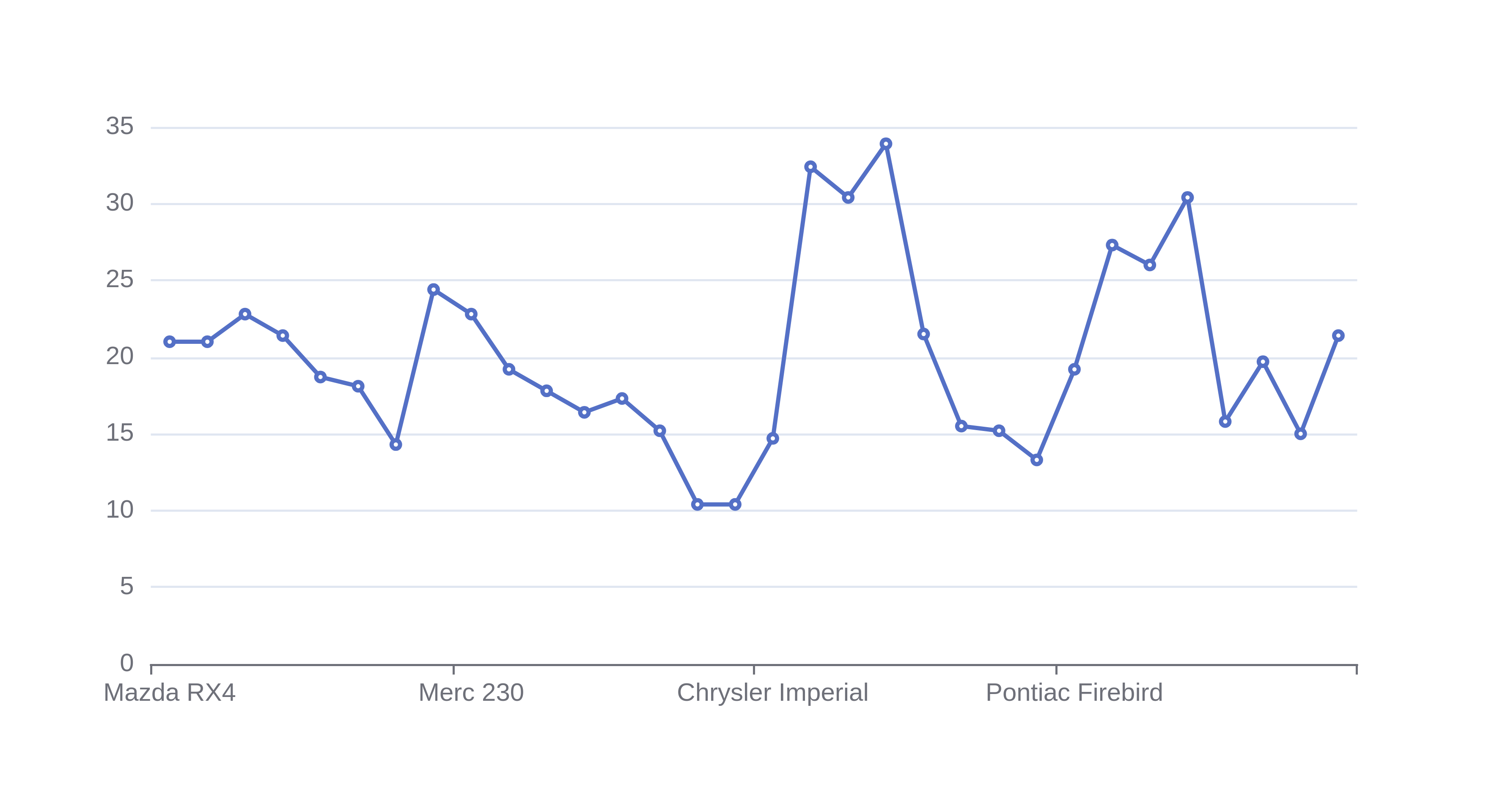# Line Graph Maker

Or input your data as csv

Sharing helps us build more free tools

Line graphs (also known as line charts) are useful for showing trends in data sets. They use a qualitative value on the y axis and can have a qualitative or quantitive value on the x axis.The line between points in a line graph do not represent a mathematical fit or average. They just join points together. This means that you cannot approximate a value between two points by looking at the line. Regression is more useful than this line graph maker if you want to interpolate to get values between points.

## When To Use aLine Graph

Use a line graph when you want to show a trend. The line between points makes it clear to readers that there is a trend between consecutive points.

If the trend is smooth then you can use a spline graph. Spline graphs are similar to line graphs, except they use a smooth line between data values.

A stacked line graph can be useful if you have multiple series of data values that add should together. For example, if you are plotting reasons why users canceled their subscriptions you might have one line for the data values of users that canceled because they did not need the product anymore and one line for the data values of users that canceled because they found another tool.

Line graphs can become really hard to read if there is a lot of data, or the trend is not very clear. In these cases, a scatter plot can be a more useful tool.

Line charts do not quantify trends in the data. Trends can be quantified using regression or correlation. Regression is usually more useful because it shows how one variable affects the other. While correlation just indicates whether there is a relationship.

## How To Make a Line Graph

2. ### Select the field for the horizontal x axis

Select the independent variable for the horizontal axis. The independent variable should not be affected by the dependent variable

3. ### Select the column for the vertical axis

Select the dependent variable for the vertical y-axis. The dependent variable should be affected by the value of the independent variable on the x-axis.